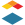# New Quizzes: Use formulas to generate multiple-choice answers

Use formulas to generate multiple-choice answers: one correct formula for the correct choice and use incorrect formulas to generate incorrect choices.

For math, physics, and related courses, the formula question option, though limited, seems to be the best tool available to generate unique, randomized, student-specific tests that can be tracked online posting (we still desperately need the option to have multiple formula answers fields in a single question). However, a simple mistake in a formula question can cost the entire points because students will have no idea what the possible answer would be. Therefore, it seems unfair to ask such a question if the instructor is not giving partial credit. One way to avoid this would be to allow multiple choices but then the problem does not become a formula question. It would really help if we can not only generate the correct answer, but also choices of incorrect answers.

One of the most frustrating issues in online exams is cheating and the technology to assist cheating seems to be moving faster than to prevent it. Therefore, it is a very pressing issue.Community Member

I am a HS Physics teacher and was hoping that someone had already brought this up. Unfortunately, there doesn't seem to be much discussion.

Creating a correct answer and three distractors would not be difficult since we should know the most common maths errors that students will generate. Allowing us to flip a fraction, forget a root, or add instead of subtract will make the questions not only more secure, but also allow for a more efficient method of review and enrichment.Community Member

I agree!

One feature that would be very helpful would be the ability to use formulas (like the formula questions) but to have a multiple choice answer format.

For example:

Question:

A person walks to the store traveling at {3 m/s} for {7.2 s}. How far did the person travel during the {7.2 s}?

The 3m/s and 7.2 s are variables with defined ranges and decimals.

The formula: answer = {3 m/s}*{7.2 s}

formula: distractor1 = answer * {0.3}     (0.3 is a variable with a defined range)

formula: distractor2 = answer * {1.9}       (1.9 is a variable with a defined range)

formula: distractor 3 = answer + {2.4}       (2.4 is a variable with a defined range)

Student view:

A person walks to the store traveling at 3 m/s for 7.2 s. How far did the person travel during the 7.2 s?

A) 21.6 m

B) 6.5 m

C) 41.0 m

D) 24 m

Students look to see if their answer of 21.6 m is an option.

This type of question format would allows students to check their answer with the possible answer choices to see if any of them match what they did. If one matches, it gives the student confidence that they did the calculations correctly. If none of the answers match, the student knows to recheck their work for a possible numerical error or conceptual one.

This also allows the students to transition into the harder, formula based questions with an numerical response.

Furthermore, with the formulas, each student can have their own dynamically created question so that students can't share numerical answers.

I think this type of option would benefit a lot of math and mathematical science classes.

Thanks,

SeanCommunity Member

100% agree.  I have to use Mastering Physics to do this right.  This is one thing that Mastering does well but it has other significant limitations. This is a must-have feature for quizzes on Canvas.Instructure
Status changed to: Archived